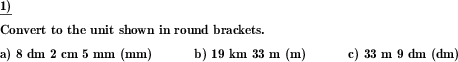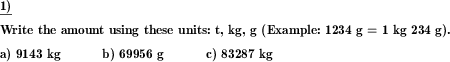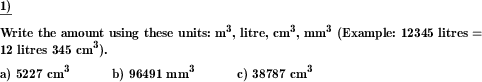Custom math worksheets at your fingertipsDetails for problem "Convert units of measurement"

Quickname: 4491

Elementary School, Primary School, Junior High School, Middle School.

Summary

Convert volume, length, time, area, time units.

ExamplesDescription

Units of measurement of different physical quantities have to be converted from a base notation covering only one unit to the proper notation with multiple units if appropriate.

The physical quantities available are

• Money (Euro, Cent)
• Money (dollars, cents)
• Length (metric units)
• Volume (metric units, litres)
• Volume (metric units, dm^3)
• Time (hms)
• Time (dhms)
• Weight (metric units)

The number of problems is adjustable. A limit can be set on the number of units covered (for example, for length, if two units are selected, the measurement may be given in m and dm, cm and mm or km and m).

The direction of conversion may be

• from a base unit to multiple units e.g. 1234mm = 1m 2dm 3cm 4mm
• or the other way round, from multiple units to a base unit, e.g. 2km 2m = 2002m.

Download free printable worksheets for this math problem here. The worksheet contains the problems only, the solution sheet includes the answers. Just click on the respective link.

•Worksheet 1Solution sheet with answers
•Worksheet 2Solution sheet with answers
•Worksheet 3Solution sheet with answers

If you can not see the solution sheets for download, they may be filtered out by an ad blocker that you may have installed. If this is the case, please allow ads for this page and reload the page. The solution sheets will then reappear.

• Do these sample worksheets do not really fit?
• Do you need more math worksheets, with a different level of difficulty?
• Would you like to combine different problems on a worksheet and adjust them to your needs?
• As a teacher, you can put together your own worksheets using the automatically generated math problems provided.
With a free initial credit, you can start creating your own math worksheets in a few minutes.

You can try it for free! Register here, to create custom worksheets now!

Customization options for this problem

Parameter
Possible values
Number of problems
1, 2, 3, 4, 5, 6, 7, 8, 9, 10
Physical quantity
Area (metric), Length (metric), Weight (metric), Time (dhms), Time (hms), Volume litres (metric), Volume dm2 (metric), Money (USD), Money (EUR)
Direction of conversion
units to base, base to units
Max number of units
2, 3, 4, 5, 6

Similar problems

Remark
Description
Convert to decimal notation and vice-versa
Write volume, length, time, area, time quantities in units and with decimals.Deutsche Version dieser Aufgabe
These informational pages with samples describe math problems that can be combined on custom math worksheets with solutions for home and K-12 school use.
Deutsche Seiten
×